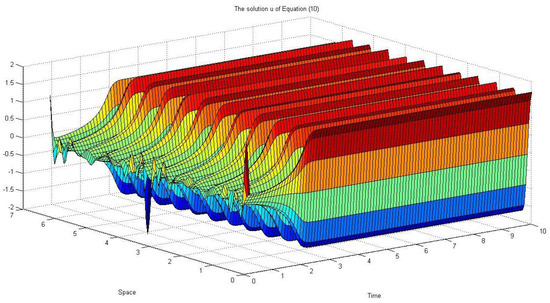Previous Article in Journal
A Fractional Equation with Left-Sided Fractional Bessel Derivatives of Gerasimov–Caputo Type
Open AccessArticle

# Modulation Equation for the Stochastic Swift–Hohenberg Equation with Cubic and Quintic Nonlinearities on the Real Line

byWael W. Mohammed 1,21
Department of mathematics, Faculty of Science, Mansoura University, Mansoura 35516, Egypt
2
Mathematics Department, Faculty of Science, University of Ha’il, Ha’il 2440, Saudi Arabia
Mathematics 2019, 7(12), 1217; https://doi.org/10.3390/math7121217
Received: 17 November 2019 / Revised: 2 December 2019 / Accepted: 7 December 2019 / Published: 10 December 2019
The purpose of this paper is to rigorously derive the cubic–quintic Ginzburg–Landau equation as a modulation equation for the stochastic Swift–Hohenberg equation with cubic–quintic nonlinearity on an unbounded domain near a change of stability, where a band of dominant pattern is changing stability. Also, we show the influence of degenerate additive noise on the stabilization of the modulation equation. View Full-Text
Show FiguresFigure 1

MDPI and ACS Style

Mohammed, W.W. Modulation Equation for the Stochastic Swift–Hohenberg Equation with Cubic and Quintic Nonlinearities on the Real Line. Mathematics 2019, 7, 1217.